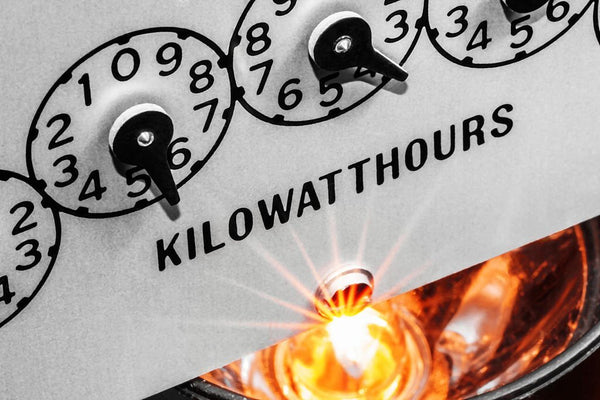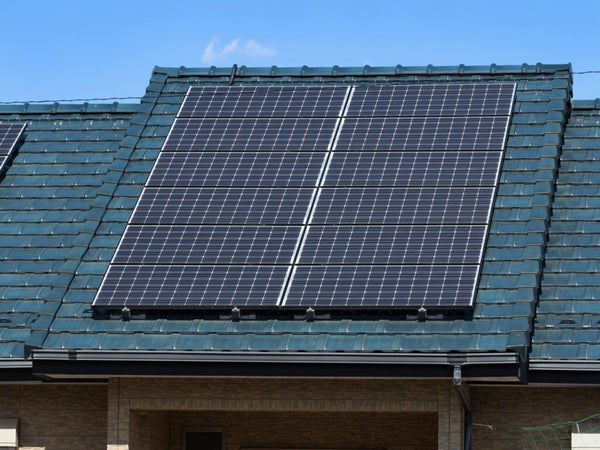Convert kWh to MWh - Understanding the Conversion Process - ShopSolar.com

# Convert kWh to MWh

The conversion process between kWh and MWh is a simple one. The complexities show up when we don’t understand what the units of power are used for.

Once you know what each unit is used for and how you derive it, converting one to another is as easy as pie.

## Understanding Kilowatt-HoursWe use kWh usage calculators to help scale our solar system. If your monthly power bill is continuously rising due to all the appliances you’re powering, then it’s best to take the solar route.

We must distinguish between kilowatts and kilowatt hours.

Kilowatts (kW) are a measure of the amount of energy a piece of equipment either uses or produces at a specific point. Kilowatt-hours (kWh) indicate how much of that energy is built up over time.

For example, a toaster will likely have a higher kilowatt rating than a fridge, but since your fridge is running pretty much the entire day, it’s going to use more kWh to stay powered.

So when we use a kW to kWh calculator, we’re simply looking at the amount of energy used over time.

### kW and W

You often see a kilowatt or watt rating on an appliance’s packaging, describing how much power is needed to use the appliance.

If we solely want to use kilowatts as our unit of measurement in calculations, but the appliance is measured in watts, we can do some simple kW to watts conversions.

### kWh

Kilowatt-hours factor in time as a variable. We use the energy formula to obtain the kilowatt hours of an appliance or household.This formula states that energy is equivalent to multiplying power by time. So, let’s say we have a heater that’s rated at 2 kW, and we keep it running for 4 hours.

If we follow our formula, we’re left with 6 kWh of energy being consumed.

Now that we’re clued up with kWh, using a kWh cost calculator or sizing system for our solar needs is less complicated.

## Understanding Megawatt-Hours

After diving into kilowatts and watts, we can say that power is the rate of energy that’s transferred and is measured in watts.

If 1,000 W is equivalent to 1 kW, then 1,000 kW is a single megawatt. This is a large amount of power usually used when dealing with industrial amounts of electricity.

Chances are you will hear megawatts come up in a conversation when people speak about the rated capacity of a generation unit.

That value mentioned in megawatts refers to the maximum instantaneous output. But what about megawatt hours?

### MWh

One megawatt hour is one megawatt of power being sustained for one hour.

In solar power generation units, chances are you will need a battery to store the energy.

This means that both megawatts and megawatts hours are used to describe the capacity of batteries for larger solar power system kits.But now that we’ve understood the variables we’re working with, how do we go about converting one to the other?

## How to convert kWh to MWh

In the same way that a single megawatt is equal to 1,000 kW, 1 MWh is equivalent to 1,000 kWh.

If you want to build a solar system made up of many arrays of solar panels, you use a kWh per square foot calculator to size the system adequately.

However, the bigger the land, the smaller you want the values you’re working with to be.

This means you can convert your kWh to MWh and use an MWh per square foot calculator instead.

## ConclusionWhen it comes to converting one unit of power to another, in most cases you would rely on laws or calculations.

However, when it comes to kW to MW, or kWh to MWh, it’s simply a matter of multiplying or dividing by 1,000 to get the unit you’re looking for.

Did You Find Our Blog Helpful? Then Consider Checking: